# College Physics Reasoning and Relationships

Physics & Astronomy

## Quiz 11 : Harmonic Motion and ElasticityStudy FlashcardsLooking for Introductory Physics Homework Help?

## Quiz 11 :Harmonic Motion and Elasticity

Question TypeWhich is not an example of approximate simple harmonic motion?
Free
Multiple Choice

A

Tags
Choose question tagDoubling the weight suspended vertically from a coil spring will result in a change in the displacement of the spring's lower end by what factor?
Free
Multiple Choice

C

Tags
Choose question tagA tiny spring, with a spring constant of 1.20 N/m, will be stretched to what displacement by a 0.0060-N force?
Free
Multiple Choice

B

Tags
Choose question tagConsider the curve x = A sin(kt), with A > 0. At which point on the graph is it possible that t = 0?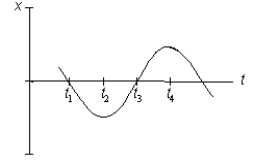Multiple Choice
Tags
Choose question tagIf it takes 8.0 N to stretch a spring 6.0 cm, and if the spring is then cut in half, what force does it take to stretch one of the halves 3.0 cm?
Multiple Choice
Tags
Choose question tagA pendulum on the Earth has a period T. The acceleration due to gravity on Mars is less than that on the Earth, and the acceleration due to gravity on the Moon is even less. Where would the period of an identical pendulum be the least?
Multiple Choice
Tags
Choose question tagIf a simple harmonic motion is described by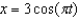, starting from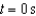, what is the first occurrence of a value of t for which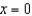? Assume SI units.
Multiple Choice
Tags
Choose question tagThe position of a 0.64-kg mass undergoing simple harmonic motion is given by x = (0.160 m) cos (t/8). What is its period of oscillation?
Multiple Choice
Tags
Choose question tagA large spring requires a force of 100 N to compress it only 0.010 m. What is the spring constant of the spring?
Multiple Choice
Tags
Choose question tagA mass of 0.40 kg, attached to a spring with a spring constant of 80 N/m, is set into simple harmonic motion. What is the magnitude of the acceleration of the mass when at its maximum displacement of 0.050 m from the equilibrium position?
Multiple Choice
Tags
Choose question tagA 0.20-kg object is attached to a spring with spring constant k = 10 N/m and moves with simple harmonic motion over a horizontal, frictionless surface. At the instant that it is displaced from equilibrium by 0.060 m, what is its acceleration?
Multiple Choice
Tags
Choose question tagIf a simple harmonic motion is described by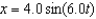, what is the period of oscillation and what is the maximum speed during the oscillation? Assume SI units.
Multiple Choice
Tags
Choose question tagSuppose there is an object for which F = +kx. What will happen if the object is moved away from equilibrium (x = 0) and released?
Multiple Choice
Tags
Choose question tagThe SI base units for the spring constant are which of the following?
Multiple Choice
Tags
Choose question tagA mass of 4.0 kg, resting on a horizontal, frictionless surface, is attached on the right to a horizontal spring with spring constant 30 N/m and on the left to a horizontal spring with spring constant 50 N/m. If this system is moved from equilibrium, what is the effective spring constant?
Multiple Choice
Tags
Choose question tagA mass on a spring vibrates in simple harmonic motion at a frequency of 4.0 Hz and an amplitude of 8.0 cm. If a timer is started when its displacement from equilibrium is a maximum (hence x = 8 cm when t = 0), what is the displacement of the mass when t = 3.7 s?
Multiple Choice
Tags
Choose question tagThe position of a 0.64-kg mass undergoing simple harmonic motion is given by x = (0.160 m) cos (t/16). What is its position at t = 6.0 s?
Multiple Choice
Tags
Choose question tagThree identical springs, each with spring constant k, are attached in parallel to a mass, which is then set into simple harmonic motion. What would be the spring constant of a single spring which would result in the same frequency of oscillation as the parallel springs?
Multiple Choice
Tags
Choose question tagDoubling the displacement from equilibrium of an object in simple harmonic motion will bring about a change in the magnitude of the object's acceleration by what factor?
Multiple Choice